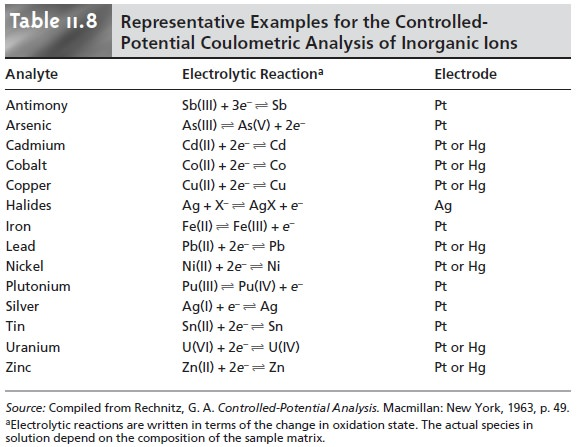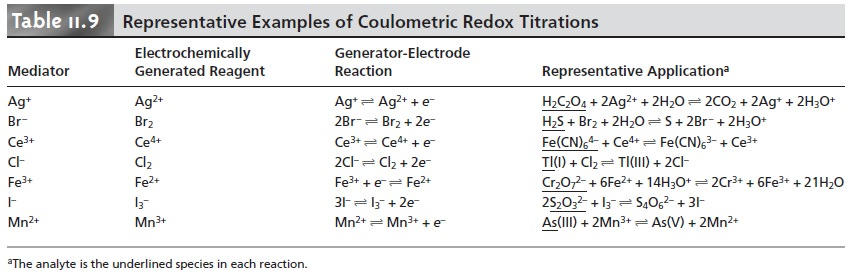Home | | Modern Analytical Chemistry | Quantitative Applications - Coulometric Methods of Analysis

# Quantitative Applications - Coulometric Methods of Analysis

Coulometry may be used for the quantitative analysis of both inorganic and organic compounds.

Quantitative Applications

Coulometry may be used for the quantitative analysis of both inorganic and organic compounds. Examples of controlled-potential and controlled-current coulometric methods are discussed in the following sections.

## Controlled-Potential Coulometry

The majority of controlled-potential coulometric analyses involve the determination of inorganic cations and anions, including trace metals and halides. Table 11.8 provides a summary of several of these methods.The ability to control selectivity by carefully selecting the working electrode’s potential, makes controlled-potential coulometry particularly useful for the analysis of alloys. For example, the composition of an alloy containing Ag, Bi, Cd, and Sb can be determined by dissolving the sample and placing it in a matrix of 0.2 M H2SO4. A platinum working electrode is immersed in the solution and held at a constant potential of +0.40 V versus the SCE. At this potential Ag(I) deposits on the Pt electrode as Ag, and the other metal ions remain in solution. When electrolysis is complete, the total charge is used to determine the amount of silver in the alloy. The potential of the platinum electrode is then shifted to –0.08 V versus the SCE, depositing Bi on the working electrode. When the coulometric analysis for bismuth is complete, antimony is determined by shifting the working electrode’s potential to –0.33 V versus the SCE, depositing Sb. Finally, cadmium is determined following its electrodeposition on the Pt electrode at a potential of –0.80 V versus the SCE.

Another area where controlled-potential coulometry has found application is in nuclear chemistry, in which elements such as uranium and polonium can be determined at trace levels. For example, microgram quantities of uranium in a medium of H2SO4 can be determined by reducing U(VI) to U(IV) at a mercury working electrode.

Controlled-potential coulometry also can be applied to the quantitative analy- sis of organic compounds, although the number of applications is significantly less than that for inorganic analytes. One example is the six-electron reduction of a nitro group, –NO2, to a primary amine, –NH2, at a mercury electrode. Solutions of picric acid, for instance, can be analyzed by reducing to triaminophenol.Another example is the successive reduction of trichloroacetate to dichloroac- etate, and of dichloroacetate to monochloroacetate

Cl3CCOO(aq)+ H3O+(aq)+ 2e < = = = = > Cl2HCCOO(aq)+ Cl(aq)+ H2O(l)

Cl2HCCOO(aq)+ H3O+(aq)+ 2e < = = = = > ClH2CCOO(aq)+ Cl(aq)+ H2O(l)

Mixtures of trichloroacetate and dichloroacetate are analyzed by selecting an initial potential at which only the more easily reduced trichloroacetate is reduced. When its electrolysis is complete, the potential is switched to a more negative potential at which dichloroacetate is reduced. The total charge for the first electrolysis is used to determine the amount of trichloroacetate, and the difference in total charge be- tween the first and second electrolyses gives the amount of dichloroacetate.

## Controlled-Current Coulometry

The use of a mediator makes controlled-current coulometry a more versatile analytical method than controlled-potential coulome- try. For example, the direct oxidation or reduction of a protein at the working elec- trode in controlled-potential coulometry is difficult if the protein’s active redox site lies deep within its structure. The controlled-current coulometric analysis of the protein is made possible, however, by coupling its oxidation or reduction to a medi- ator that is reduced or oxidized at the working electrode. Controlled-current coulo- metric methods have been developed for many of the same analytes that may be de- termined by conventional redox titrimetry. These methods, several of which are summarized in Table 11.9, also are called coulometric redox titrations.Coupling the mediator’s oxidation or reduction to an acid–base, precipitation, or complexation reaction involving the analyte allows for the coulometric titration of analytes that are not easily oxidized or reduced. For example, when using H2O as a mediator, oxidation at the anode produces H3O+

6H2O(l) < = = = = > 4H3O+(aq)+ O2(g)+ 4e

while reduction at the cathode produces OH.

2H2O(l)+ 2e < = = = = > 2OH(aq)+ H2(g)

If the oxidation or reduction of H2O is carried out externally using the generator cell shown in Figure 11.25, then H3O+ or OH can be dispensed selectively into a solution containing a basic or acidic analyte. The resulting reaction is identical to that in an acid–base titration. Coulometric acid–base titrations have been used for the analysis of strong and weak acids and bases, in both aqueous and nonaqueous matrices.Examples of coulometric titrations involving acid–base, complexation, and precipitation reactions are summarized in Table 11.10.In comparison with conventional titrimetry, there are several advantages to the coulometric titrations listed in Tables 11.9 and 11.10. One advantage is that the electrochemical generation of a “titrant” that reacts immediately with the analyte al- lows the use of reagents whose instability prevents their preparation and storage as a standard solution. Thus, highly reactive reagents such as Ag2+ and Mn3+ can be used in coulometric titrations. Because it is relatively easy to measure small quantities of charge, coulometric titrations can be used to determine small quantities of analyte that cannot be measured accurately by a conventional titration.

## Quantitative Calculations

The absolute amount of analyte in a coulometric analy- sis is determined by applying Faraday’s law (equation 11.23) with the total charge during the electrolysis given by equation 11.24 or equation 11.25. Example 11.8 shows the calculations for a typical coulometric analysis.## Representative Method

Every controlled-potential or controlled-current coulo- metric method has its own unique considerations. Nevertheless, the following pro- cedure for the determination of dichromate by a coulometric redox titration pro- vides an instructive example.

Study Material, Lecturing Notes, Assignment, Reference, Wiki description explanation, brief detail
Modern Analytical Chemistry: Electrochemical Methods of Analysis : Quantitative Applications - Coulometric Methods of Analysis |